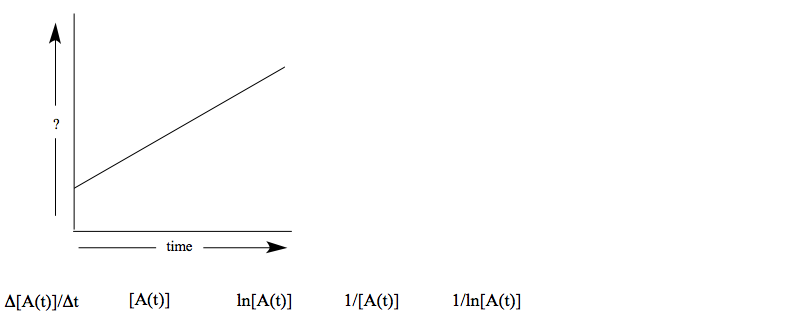# Problem: Below is a plot of an integrated rate law vs. time. Which of the following could be the correct label for the y-axis? (Circle one)

###### FREE Expert Solution
100% (196 ratings)
###### Problem Details

Below is a plot of an integrated rate law vs. time. Which of the following could be the correct label for the y-axis? (Circle one)